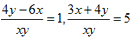Wednesday 19th February 2020CBSE Guess > Papers > Important Questions > Class X > 2014 > Mathematics > Math-index > Mathematics By Mr. Ajai Kumar Shukla CBSE CLASS X Mathematics CLASS X MATHS (H.W) Use Euclid’s division lemma to show that the square of any positive integer of the form 3m or (3m+1) for some integer m. Prove that 2 - 3√5 is an irrational number. On dividing x3 - 3x2 + x + 2 by a polynomial g(x), the quotient & the remainder are x-2  &  - 2x + 4 respectively. Find g(x). Find the value of k for which the polynomial x4 +10x3 +25x2+15x+k is exactly divisible by x+7. For what value of x will x4 - 3x3 + 7x2 - 8x+9 be exactly divisible by x2-x+4? If the polynomial x4 - 6x3 + 16x2 - 25x +10 is divided by another polynomial x2 - 2x+k the remainder comes out to be x + a. Find the values of k & a. Graphically find whether the pair of linear equations: 4x – 3y + 5 = 0  ; 6x-4.55 +7.5=0 is consistent or inconsistent. Solve :Solve:  10/(x +y) +4/(y –x) = -2 , 15/(x +y ) – 7/(y –x) =10 A number consists of two digits. When it is divided by the sum of the digits, the quotient is 7.The sum of the reciprocals of the digits is 9 times the product of the reciprocals of the digits. Find the number. 2 men & 5boys can do a piece of work in 4 days while 4 men & 4 boys can do it in 3 days. How long would it take one man or one boy to do it? Taxi fare consists of a constant charge together with the charge for the distance covered. A person travelling 15km pays Rs115 for the journey & a person travelling 27km pays Rs199 for it. Find the charges one will have to pay for a journey of 50 km. A boat goes 30km upstream & 44km downstream in 10hrs. It can go 40km upstream & 55km downstream in 13hrs. Find the speed of the stream & that of boat in still water. Meena went to bank to withdraw Rs2000.She asked the cashier to give her Rs50 & Rs100 notes only. If Meena got 25 notes in all, find how many notes of Rs50 & Rs100 she got. Prove each of the following: (sec A – cos A) (cot A + tan A) = tan A secA (1 + cot A – cosec A)(1 + tan A + sec A) = 2 tan2 A + cot2 A + 2 = sec2 Acosec2A sec4 A – sec2A = tan4 A + tan2A CBSE Important Questions Class X Mathematics By : Mr. Ajai Kumar Shukla   Submitted By : Mr. Ajai Kumar Shukla, RACHNA TUTORIALS, 6/1003 JANKIPURAM VISTAR LUCKNOW Mobile : +91 - 9415467421 Email Id : [email protected]